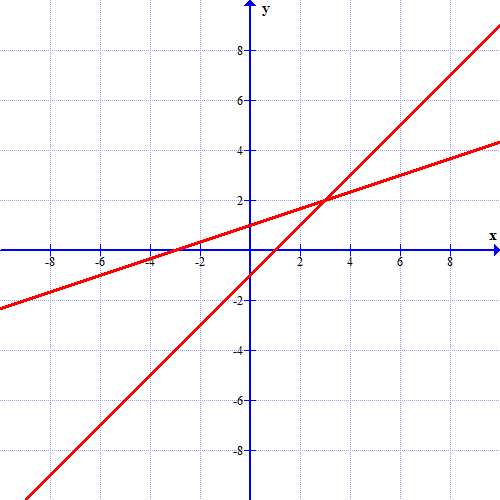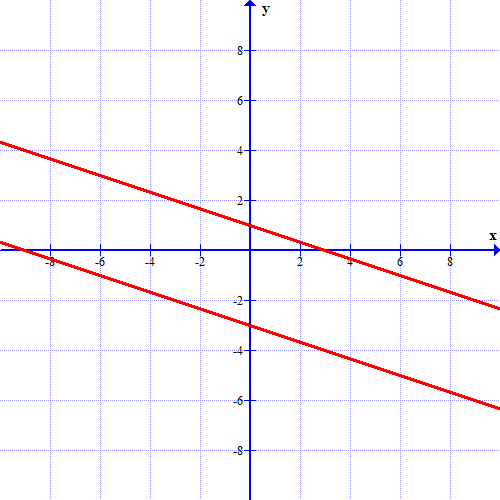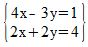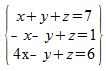# FTCE Middle Grades Math: Systems of Linear Equations Chapter Exam

Exam Instructions:

Choose your answers to the questions and click 'Next' to see the next set of questions. You can skip questions if you would like and come back to them later with the yellow "Go To First Skipped Question" button. When you have completed the practice exam, a green submit button will appear. Click it to see your results. Good luck!

### Page 1

#### Question 4 4. Look at the graph. What is the solution to the system of equations?### Page 2

#### Question 6 6. Which variable or variables need to be eliminated from the third equation in order to solve via the Gaussian elimination method?#### Question 9 9. What is the solution to the system of equations shown in the graph below?### Page 3

#### Question 13 13. Solve the following system of equations.#### Question 14 14. What can you do to eliminate the x in the second equation?### Page 5

#### Question 25 25. Which variable in which equation needs to be eliminated in order to solve this system using Gaussian elimination?#### FTCE Middle Grades Math: Systems of Linear Equations Chapter Exam Instructions

Choose your answers to the questions and click 'Next' to see the next set of questions. You can skip questions if you would like and come back to them later with the yellow "Go To First Skipped Question" button. When you have completed the practice exam, a green submit button will appear. Click it to see your results. Good luck!

Support